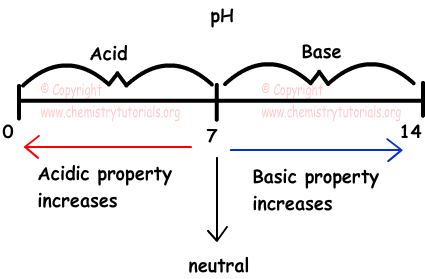## pH pOH and Ionization of Water

Ionization of Water

Water ionize as given below;

H2O(l) ↔ H+(aq) + OH-(aq)

In pure water concentrations of H+ and OH- ions are equal to each other and at 25 0C, they have concentration 1x10-7 M. Since concentration of ion in pure water is too  low, it is a bad electric conductor.

As in the case of pure water mediums having [H+]=[OH-] concentration are called neutral mediums. In water solutions multiplication of [H+] and [OH-] is constant and at 25 oC it is 1x10-14. This number is also called ionization constant of pure water.

If acid is added to pure water;

[H+]>1x10-7 M and [OH-]<1x10-7

If base is added to pure water;

[OH-]>1x10-7 M and [H+]<1x10-7

To sum up we can say that;

• If concentration of  [H+] = [OH-]=10-7M, then solution is neutral.
• If concentration of  [H+] > [OH-] or [H+]>10-7M and [OH-]<10-7 M, then solution is acidic.
• If concentration of  [OH-]>[H+] or [H+]<10-7M and [OH-]>10-7 M, then solution is basic.

Example: HCl having volume 224 cm3 under standard conditions mixed with pure water and form 1 L solution. Which ones of the following statements are true for this solution?

I. Concentration of solution is 10-2 molar.

II. Concentration of H+ ion is 10-2 molar.

III. Concentration of OH- ion is 10-12 molar.

Solution:

We find mole of HCl  gas under standard conditions.

1 mole gas has volume 22,4 L and 1 L= 1000 cm3

nHCl=224/22400=0,01 mol

Molarity of HC solution is;

[HCl]=0,01/1=0,01 M or [HCl]=10-2 M I is true

Since HCl is strong acid, it is completely ionize in solution. Thus, concentration of H+ ion is equal to concentration of HCl. II is true.

[H+].[OH-]=10-14

10-2.[OH-]=10-14

[OH-]=10-12 molar III is true.

pH and pOH

In liquid solutions, to state concentrations of H+ and OH- ions pH and pOH concepts are used. We can show pH and pOH in terms of concentration as;

pH=-log[H+]

and

pOH=-log[OH-]

Solution having molar concentration of H+=10-2 M has pH=2 and solution having molar concentration of OH-=10-5 has pOH=5. To remember logarithmic calculations;

[H+].[OH-]=10-14 log of this equation;

log[H+] + log[OH-]=-14

-log[H+] - log[OH-]=14

pH + pOH = 14

In acid solutions, [H+]>10-7 or pH<7

In base solutions [H+]<10-7 or pH>7

• If 7>pH>0 acidic solution
• If 14>pH>7 basic solution
• If pH=7 neutral solution

Picture given below summarizes what we try to explain above;Example: Which ones of thee following statements are true for water solutions?

I. If pH=pOH=7 , then solution is neutral

II. If [H+]>10-7 then pH<7

III. If [OH-]>[H+] then pH<7

Solution:

I is true is pH=pOH=7

If [H+]>10-7 M then pH<7  II is true

If [OH-]>[H+] then pH>7 III is false.

Acids and Bases Exams and  Problem Solutions

Author:

© Copyright www.ChemistryTutorials.org, Reproduction in electronic and written form is expressly forbidden without written permission of www.ChemistryTutorials.org. Privacy Policy